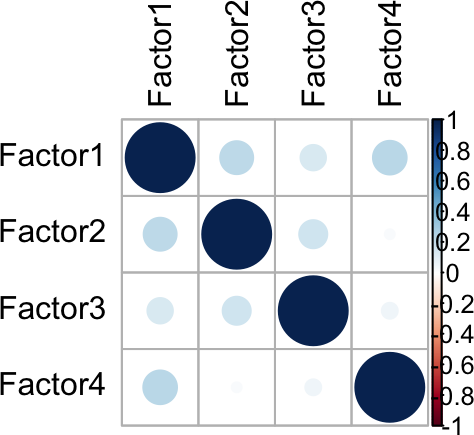``````library(MOFA2)
library(tidyverse)
library(cowplot)
library(magrittr)``````

# 1 Load ST data set

We use the example data provided in the Seurat vignette, which contains spatial transcriptomics data on a slide of a mouse brain tissue generated using 10x visium.

``library(Seurat)``
``````## Registered S3 method overwritten by 'spatstat':
##   method     from
##   print.boxx cli``````
``SeuratData::InstallData("stxBrain")``
``````## Warning: The following packages are already installed and will not be
## reinstalled: stxBrain``````
``````brain <- SeuratData::LoadData("stxBrain", type = "anterior1")
brain <- Seurat::NormalizeData(brain, assay = "Spatial", verbose = FALSE)
brain <- Seurat::FindVariableFeatures(brain, assay = "Spatial")``````

# 2 Preprocess data for MEFISTO

Next, we prepare the data for input to MEFISTO. For this we need the expression data (using the 2000 top variable features) as well as the spatial coordinates of each sample (spot).

``````expression_data <- as.matrix(brain@assays\$Spatial@data[VariableFeatures(brain),])

dim(expression_data)``````
``##  2000 2696``
``````locs <- GetTissueCoordinates(brain)
locs <- tibble::rownames_to_column(locs, "sample") %>%
gather(key = "covariate", value = "value", starts_with("image"))

``````##               sample covariate    value
## 1 AAACAAGTATCTCCCA-1  imagerow 385.9725
## 2 AAACAAGTATCTCCCA-1  imagecol 438.9501
## 3 AAACACCAATAACTGC-1  imagerow 441.6351
## 4 AAACACCAATAACTGC-1  imagecol 143.9587
## 5 AAACAGAGCGACTCCT-1  imagerow 163.3735
## 6 AAACAGAGCGACTCCT-1  imagecol 410.4991``````

# 3 Create a MOFA object specifying spatial coordinates as covariates

To create a space-aware MOFA model (= MEFISTO) we provide MOFA the expression data as well as the spatial coordinates in `set_covariates`.

``````# create MOFA objects and specify the spatial coordinates as covariates
sm <- create_mofa(data = list(RNA = expression_data))``````
``## Creating MOFA object from a list of matrices (features as rows, sample as columns)...``
``sm <- set_covariates(sm, locs)``

# 4 Prepare training

Before training, we can specify various options for the model, the training and the data pre-processing. If no options are specified, the model will use the default options. See also `get_default_data_options`, `get_default_model_options`, `get_default_training_options` and `get_default_mefisto_options` to have a look at the various options and their defaults. Here, we in particular, specify that we want to use spare Gaussian process with a smaller number of inducing points which can speed up training.

``````model_opts <- get_default_model_options(sm)
model_opts\$num_factors <- 4

n_inducing <- 1000 # We use 1000 inducing points to learn spatial covariance patterns
mefisto_opts <- get_default_mefisto_options(sm)
mefisto_opts\$sparseGP <- TRUE
mefisto_opts\$frac_inducing <- n_inducing / get_dimensions(sm)\$N

train_opts <- get_default_training_options(sm)
train_opts\$seed <- 2021

sm <- prepare_mofa(sm, training_options = train_opts,
model_options = model_opts,
mefisto_options = mefisto_opts)``````
``## Checking data options...``
``## No data options specified, using default...``
``## Checking training options...``
``## Checking model options...``
``## Checking inference options for mefisto covariates...``

Next, we train the model. As running the model can take some time, we here load a pre-trained model. This can be found here.

``outfile = "data/ST_model.hdf5"``
``# sm <- run_mofa(sm, outfile = outfile)``

# 5 Load a trained model

``sm <- load_model(outfile)``

# 6 Downstream analyses

## 6.1 Variance decomposition

First, we can take a look whether our factor are uncorrelated.

``plot_factor_cor(sm)``## 6.2 Spatial factors

Next, we will have a look at the spatial patterns that are captured by each factor. We here rotate the tissue to obtain the same as orientation as in `SpatialFeaturePlot` below.

``plot_factors_vs_cov(sm, rotate_y = TRUE)``